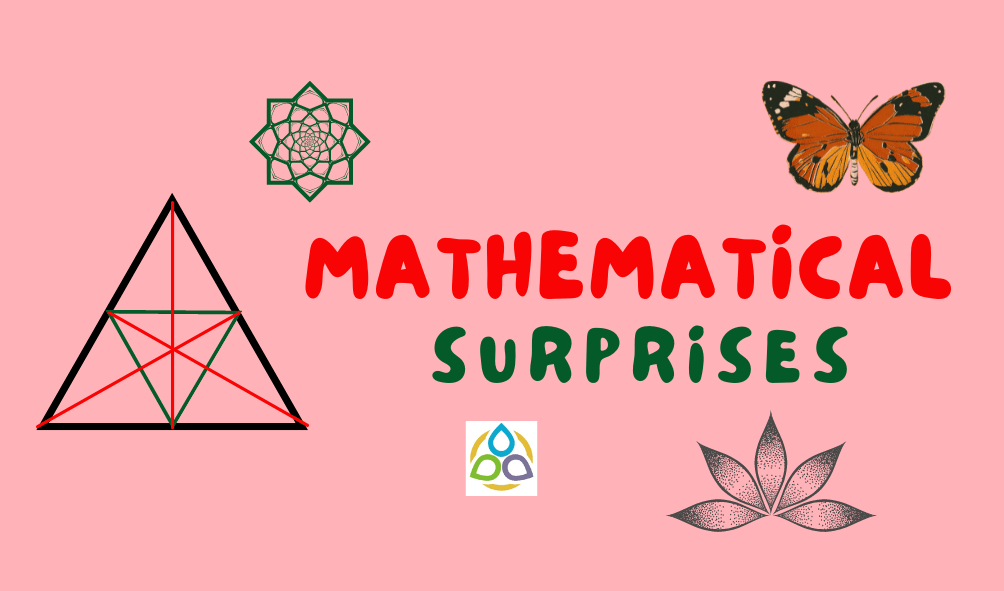# Mathematical Surprises

Mathematical coincidences are events in which two seemingly unrelated mathematical concepts, formulas or expressions end up being equal or related in an unexpected way. These coincidences can be surprising and intriguing, and they have been the subject of fascination for mathematicians for centuries. One famous example of a mathematical coincidence is Euler’s identity:

e + 1 = 0

where e is Euler’s number, i is the imaginary unit, and π is pi, the ratio of the circumference of a circle to its diameter. This formula relates five fundamental mathematical constants, each of which arises in different areas of mathematics. It is remarkable that these seemingly unrelated constants should be linked in such a simple equation.

While mathematical coincidences can be intriguing and thought-provoking, it is important to remember that they are ultimately the result of underlying mathematical relationships and patterns. As such, they are not truly “coincidences” in the sense of being random or arbitrary. Rather, they are manifestations of the deep interconnectedness of different areas of mathematics and serve as a reminder of the beauty and elegance of the subject.